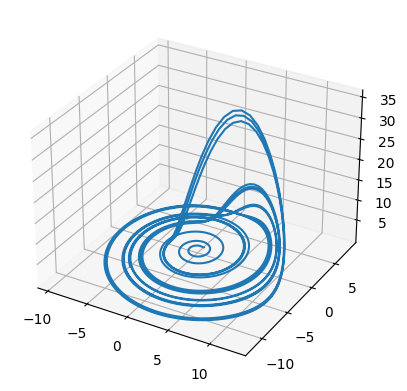# Basic Visualization with matplotlib¶

Python’s most commonly used plotting library is matplotlib. The library has an interface which mirrors that of Mathworks’ Matlab software, and so those with matlab familiarity will find themselves already high up on the learning curve.

## Loading matplotlib and setting up the notebook environment¶

The matplotlib plotting library has a magic connection with the iPython shell and the notebook environment that allows static images of plots to be rendered in the notebook. Instead of using the normal import ... syntax, we’ll use this iPython ‘magic’ to not only import the library, but set up the environment we’ll need to create plots.

%pylab inline

Populating the interactive namespace from numpy and matplotlib


We’ll use the emissions data we saw before in the Pandas tutorial, as it’s familiar:

import pandas as pd
skiprows=2, index_col='Year',
names=['Year', 'Total Emissions',
'Gas Emissions', 'Liquid Emissions',
'Solid Emissions', 'Cement Emissions',
'Flare Emissions', 'Per Capita Emissions'])


/Users/houghton/anaconda/lib/python2.7/site-packages/pandas/computation/__init__.py:19: UserWarning: The installed version of numexpr 2.4.4 is not supported in pandas and will be not be used

UserWarning)

Total Emissions Gas Emissions Liquid Emissions Solid Emissions Cement Emissions Flare Emissions Per Capita Emissions
Year
1751 3 0 0 3 0 0 NaN
1752 3 0 0 3 0 0 NaN
1753 3 0 0 3 0 0 NaN

## Basic plotting¶

The basic plot command takes as its first two arguments the x and y values of the points which we wish to plot:

plt.plot(emissions.index, emissions['Total Emissions']);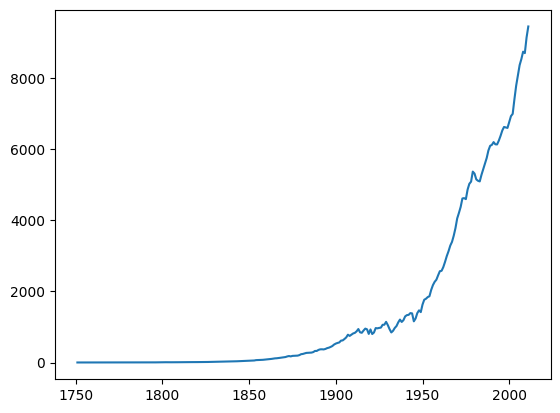## Labeling axes and title¶

Following out plot command we can submit commands to add text to the figure, such as adding labels to the x and y axes, and a title to the figure.

plt.plot(emissions.index, emissions['Total Emissions'])
plt.xlabel('Year')
plt.ylabel('Million Metric Tons CO2/Year')
plt.title('Historical CO2 Emissions', fontsize=18);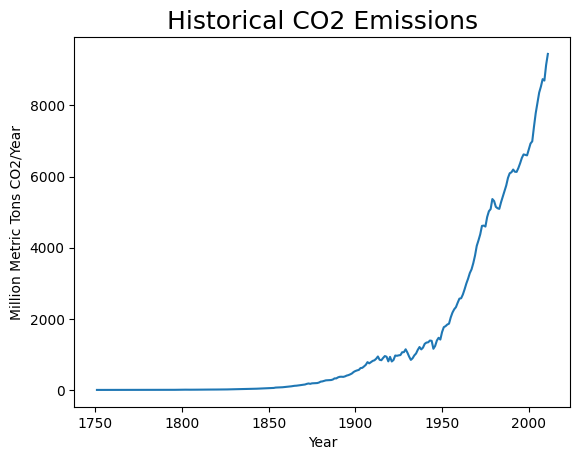## Changing line properties¶

We can include various elements into the plot command to specify how the line will look:

plt.plot(emissions.index, emissions['Total Emissions'], 'ro', alpha=.5);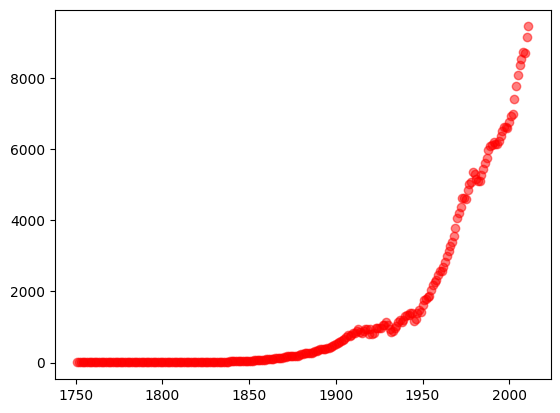## Specifying axis bounds¶

We can specify that we want our plot to be bounded by various x and y values:

plt.plot(emissions.index, emissions['Total Emissions'])
plt.xlim(1950,2000)
plt.ylim(1500,7500);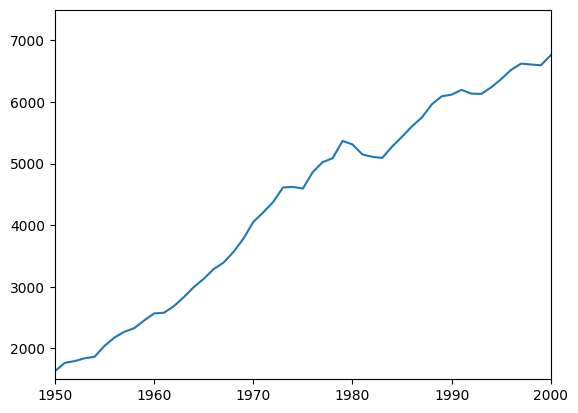## Multiple lines¶

We can add lines to our plot simply by adding additional calls to the plot function. Passing the plot function an argument called ‘label’ allows us to format a legend with appropriate references to each line:

plt.plot(emissions.index, emissions['Liquid Emissions'], 'r', label='Liquid')
plt.plot(emissions.index, emissions['Solid Emissions'], 'b', label='Solid')
plt.plot(emissions.index, emissions['Gas Emissions'], 'g', label='Gas')
plt.legend(loc='upper left');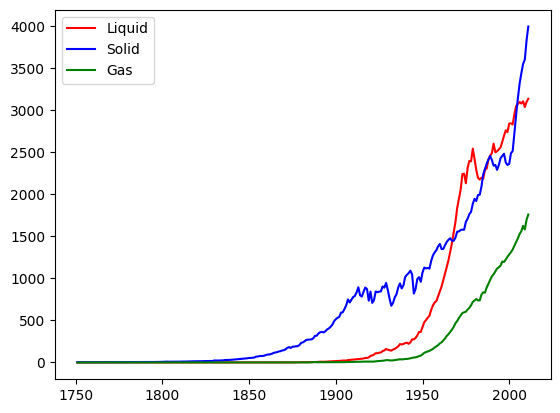## Other plot types¶

There are a number of other plot types available, such as histograms, radial plots, plots with logarithmic axes, or stackplots:

plt.stackplot(emissions.index, [emissions['Liquid Emissions'],
emissions['Gas Emissions'],
emissions['Solid Emissions']],
labels=['Liquid', 'Gas', 'Solid'])
plt.legend(loc='upper left');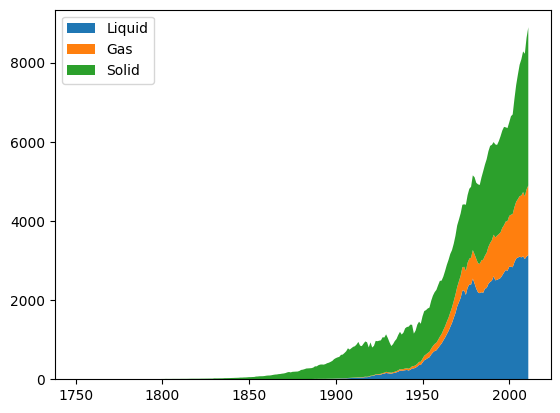## Saving figures¶

We can save a figure to the disk by calling matplotlib’s savefig function:

plt.plot(emissions.index, emissions['Total Emissions'])
plt.savefig('Figure_1_Total_Emissions.png')### Matplotlib and Pandas¶

Pandas uses matplot lib to provide a basic plotting interface of its own. The dataframe we have been working with has a convenience method called .plot(), which assumes some basic format for how you would like your data presented, and tries to do so for you.

This is handy when you are just interested in having a quick look at your data, without going to the trouble to create finished plots.

emissions.plot();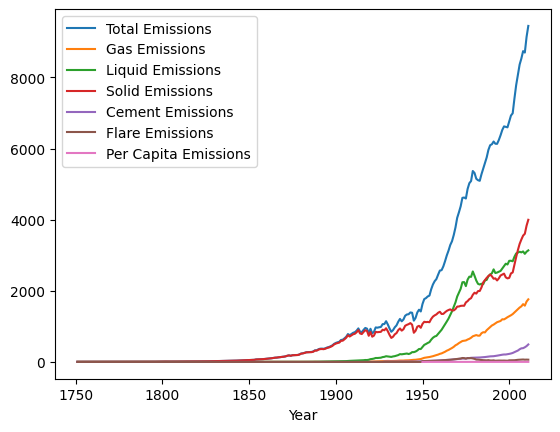The Dataframe’s wrapper of matplotlib gives us a number of basic options for how our plots are shown:

emissions.plot(subplots=True, figsize=(10,6));### Matplotlib and PySD¶

As PySD returns a Pandas Dataframe, we can either use the plotting interface directly, or Pandas’s convenience wrapper. Here we’ll load a model which produces a chaotic output in three dimensions to use in our demonstration.

import pysd
res = model.run()

x y z
0.00000 0.500000 0.500000 0.400000
0.03125 0.471875 0.518750 0.341250
0.06250 0.445000 0.536738 0.291747
0.09375 0.419110 0.553999 0.250087
0.12500 0.393982 0.570559 0.215065

Plotting vs. time.

plt.plot(res.index, res['x'], 'r')
plt.plot(res.index, res['y'], 'b')
plt.plot(res.index, res['z'], 'g');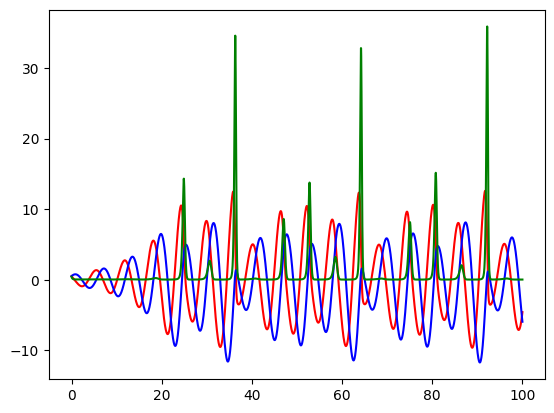Plotting variables against one another

plt.plot(res['x'], res['y']);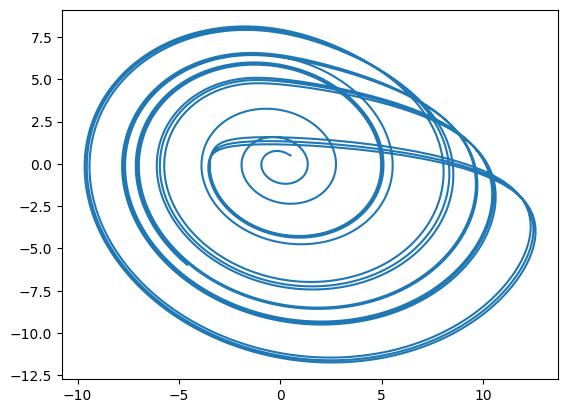While so far I have shown mostly basic, 2d plots, we can also call on matplotlib’s 3d plotting engine

import matplotlib.pyplot as plt
from mpl_toolkits.mplot3d import Axes3D
fig = plt.figure()